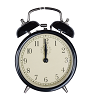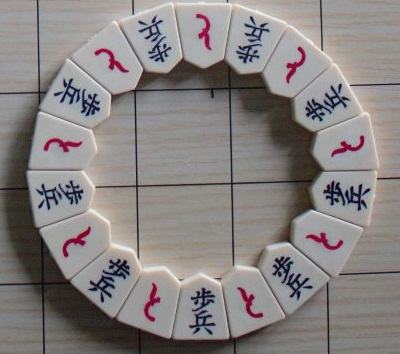#### You may also like### On Time

On a clock the three hands - the second, minute and hour hands - are on the same axis. How often in a 24 hour day will the second hand be parallel to either of the two other hands?### Estimating Angles

How good are you at estimating angles?### LOGO Challenge 1 - Star Square

Can you use LOGO to create this star pattern made from squares. Only basic LOGO knowledge needed.

# Shogi Shapes

### Why do this problem?

This problem offers an enticing context within which to work on angles and regular polygons. Unlike an exercise in which students may be invited to calculate particular angles for no apparent reason, the images in this problem are, we hope, intriguing enough to provoke students' curiosity so that they ask "I wonder what angles are needed to create this shape? I wonder if I could recreate it for myself?"

### Possible approach

Begin with the first four shapes and challenge students to work out as many different angles as they can. You may wish to print out this worksheet: Shogi Shapes.

As students are working, listen out for interesting and useful insights that they are using to work out the angles, and share them in a short plenary.

Then show this image:Invite students to come up with questions to explore based on the image. Some suggested questions are listed below. Then choose as a class which questions to work on. Finally, bring the class together to discuss what they have found out about this image.

### Key questions

Is there a quick way to count the number of tiles in the picture?
What shape are the tiles?
Which angles can be calculated?
If we assume that the tiles are symmetrical, there are two pairs of matching angles and one other angle. What is the range of possible sizes of that "lonely" angle?
Could we make other sets where we need more tiles to go all the way round with no gaps?

### Possible extension

Students could use ruler, compasses and protractors, or dynamic geometry software such as GeoGebra, to recreate the images from the problem.

### Possible support

The Angles, Polygons and Geometrical Proof short problems collection might be a useful source of questions to prepare students for this task.

The advice offered in Getting Started might also be helpful.